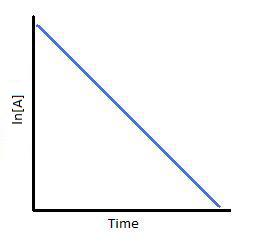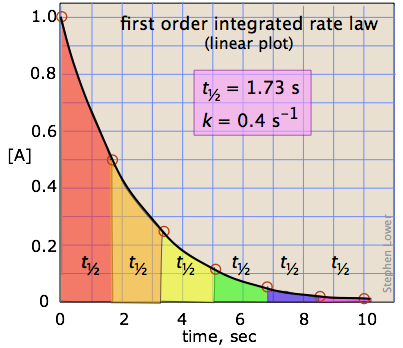# First-Order Reactions

A first-order reaction is a reaction that proceeds at a rate that depends linearly on only one reactant concentration.

## The Differential Representation

Differential rate laws are generally used to describe what is occurring on a molecular level during a reaction, whereas integrated rate laws are used for determining the reaction order and the value of the rate constant from experimental measurements. The differential equation describing first-order kinetics is given below:

$Rate = - \dfrac{d[A]}{dt} = k[A]^1 = k[A] \label{1}$

The "rate" is the reaction rate (in units of molar/time) and $$k$$ is the reaction rate coefficient (in units of 1/time). However, the units of $$k$$ vary for non-first-order reactions. These differential equations are separable, which simplifies the solutions as demonstrated below.

## The Integral Representation

First, write the differential form of the rate law.

$Rate = - \dfrac{d[A]}{dt} = k[A] \label{2}$

Rearrange to give:

$\dfrac{d[A]}{[A]} = - k\,dt \label{3}$

Second, integrate both sides of the equation.

$\int_{[A]_o}^{[A]} \dfrac{d[A]}{[A]} = -\int_{t_o}^{t} k\, dt \label{4a}$

$\int_{[A]_{o}}^{[A]} \dfrac{1}{[A]} d[A] = -\int_{t_o}^{t} k\, dt \label{4b}$

Recall from calculus that:

$\int \dfrac{1}{x} = \ln(x) \label{5}$

Upon integration,

$\ln[A] - \ln[A]_o = -kt \label{6}$

Rearrange to solve for [A] to obtain one form of the rate law:

$\ln[A] = \ln[A]_o - kt \label{7}$

This can be rearranged to:

$\ln [A] = -kt + \ln [A]_o \label{8}$

This can further be arranged into y=mx +b form:

$\ln [A] = -kt + \ln [A]_o \label{9}$

The equation is a straight line with slope m:

$mx=-kt \label{10}$

and y-intercept b:

$b=\ln [A]_o \label{11}$

Now, recall from the laws of logarithms that

$\ln {\left(\dfrac{[A]_t}{ [A]_o}\right)}= -kt \label{12}$

where [A] is the concentration at time $$t$$ and $$[A]_o$$ is the concentration at time 0, and $$k$$ is the first-order rate constant.Figure 1: Decay profiles for first-order reactions with large and small rate constants.

Because the logarithms of numbers do not have any units, the product $$-kt$$ also lacks units. This concludes that unit of k in a first order of reaction must be time-1. Examples of time-1 include s-1 or min-1. Thus, the equation of a straight line is applicable:

$\ln [A] = -kt + \ln [A]_o.\label{15}$

To test if it the reaction is a first-order reaction, plot the natural logarithm of a reactant concentration versus time and see whether the graph is linear. If the graph is linear and has a negative slope, the reaction must be a first-order reaction.

To create another form of the rate law, raise each side of the previous equation to the exponent, e:

$\large e^{\ln[A]} = e^{\ln[A]_o - kt} \label{16}$

Simplifying gives the second form of the rate law:

$[A] = [A]_{o}e^{- kt}\label{17}$

The integrated forms of the rate law can be used to find the population of reactant at any time after the start of the reaction. Plotting ln[A] with respect to time for a first-order reaction gives a straight line with the slope of the line equal to -k. More information can be found in the article on rate laws.

This general relationship, in which a quantity changes at a rate that depends on its instantaneous value, is said to follow an exponential law. Exponential relations are widespread in science and in many other fields. Consumption of a chemical reactant or the decay of a radioactive isotope follow the exponential decay law. Its inverse, the law of exponential growth, describes the manner in which the money in a continuously-compounding bank account grows with time, or the population growth of a colony of reproducing organisms. The reason that the exponential function $$y=e^x$$ so efficiently describes such changes is that dy/dx = ex; that is, ex is its own derivative, making the rate of change of $$y$$ identical to its value at any point.

## Graphing First-order Reactions

The following graphs represents concentration of reactants versus time for a first-order reaction.Plotting $$\ln[A]$$ with respect to time for a first-order reaction gives a straight line with the slope of the line equal to $$-k$$.## Half-lives of first order reactions

The half-life ($$t_{1/2}$$) is a timescale on which the initial population is decreased by half of its original value, represented by the following equation.

$[A] = \dfrac{1}{2} [A]_o$

After a period of one half-life, $$t = t_{1/2}$$ and we can write

$\dfrac{[A]_{1/2}}{[A]_o} = \dfrac{1}{2}=e^{-k\,t_{1/2}} \label{18}$

Taking logarithms of both sides (remember that $$\ln e^x = x$$) yields

$\ln 0.5 = -kt\label{19}$

Solving for the half-life, we obtain the simple relation

$t_{1/2}=\dfrac{\ln{2}}{k} \approx \dfrac{0.693}{k}\label{20}$

This indicates that the half-life of a first-order reaction is a constant.Figure 2: Half lives graphically demonstrated for first-order reaction. Notice the the half-life is independent of initial concentration. This is not the case with other reaction orders.

Example 1: Estimated Rate Constants

The half-life of a first-order reaction was found to be 10 min at a certain temperature. What is its rate constant?

SOLUTION

Use Equation 20 that relates half life to rate constant for first order reactions:

$k = \dfrac{0.693}{600 \;s} = 0.00115 \;s^{-1}$

As a check, dimensional analysis can be used to confirm that this calculation generates the correct units of inverse time.

Notice that, for first-order reactions, the half-life is independent of the initial concentration of reactant, which is a unique aspect to first-order reactions. The practical implication of this is that it takes as much time for [A] to decrease from 1 M to 0.5 M as it takes for [A] to decrease from 0.1 M to 0.05 M. In addition, the rate constant and the half life of a first-order process are inversely related.Example 2: Determining Half life

If 3.0 g of substance $$A$$ decomposes for 36 minutes the mass of unreacted A remaining is found to be 0.375 g. What is the half life of this reaction if it follows first-order kinetics?

SOLUTION

There are two ways to approach this problem: The :simple inspection approach" and the "brute force approach"

Approach #1: "The simple Inspection Approach"

This approach is used when one can recognize that the final concentration of $$A$$ is $$\dfrac{1}{8}$$ of the initial concentration and hence three half lives $$\left(\dfrac{1}{2} \times \dfrac{1}{2} \times \dfrac{1}{2}\right)$$ have elapsed during this reaction. Then use equation 18:

$t_{1/2} = \dfrac{36\, \text{min}}{3}= 12 \; \text{min}$

This approach works only when the final concentration is $$\left(\frac{1}{2}\right)^n$$ that of the initial concentration, then $$n$$ is the number of half lives that have elapsed. If this is not the case, then approach #2 can be used.

Approach #2: "The brute force approach"

This approach involves solving for $$k$$ from the integral rate law equation (Eq. 12 or 17) and then relating $$k$$ to the $$t_{1/2}$$ via Equation 20.

$\dfrac{[A]_t}{[A]_o} =e^{-k\,t}$

$k= -\dfrac{\ln \dfrac{[A]_t}{[A]_o}}{t} = -\dfrac{\ln \dfrac{0.375\, g}{3\, g}}{36\, \text{min}} = 0.0578 \, \text{min}^{-1}$

$t_{1/2}=\dfrac{\ln{2}}{k} \approx \dfrac{0.693}{0.0578 \, \text{min}^{-1}} \approx 12\, \text{min}$

The first approach is considerably faster (if the number of half lives evolved is apparent).

## Practice Problems

Calculate the half-life of the reactions below:

1. If 4.00 g A are allowed to decompose for 40 min, the mass of A remaining undecomposed is found to be 0.80 g.
2. If 8.00 g A are allowed to decompose for 34 min, the mass of A remaining undecomposed is found to be 0.70 g.
3. If 9.00 g A are allowed to decompose for 24 min, the mass of A remaining undecomposed is found to be 0.50 g.

Determine the percent H2O2 that decomposes in the time using $$k=6.40 \times 10^{-5} s^{-1}$$

1. The time for the concentration to decompose is 600.0 s after the reaction begins. Use the value of k above.
2. The time for the concentration to decompose is 450 s after the reaction begins. Use the value of k above.

## Solutions

Use the half life reaction that contains initial concentration and final concentration. Plug in the appropriate variables and solve to obtain:

1. 17.2 min
2. 9.67 min
3. 5.75 min
4. Rearranging Eq. 17 to solve for the $$[H_2O_2]_t/[H_2O_2]_0$$ ratio​ $\dfrac{[H_2O_2]_t}{[H_2O_2]_0} = e^{-kt}$ This is a simple plug and play application once you have identified this equation. $\dfrac{[H_2O_2]_{t=600\;s}}{[H_2O_2]_0} = e^{-(6.40 \times 10^{-5} s^{-1}) (600 \, s)}$ $\dfrac{[H_2O_2]_{t=600\;s}}{[H_2O_2]_0} = 0.9629$ So 100-96.3=3.71% of the hydrogen peroxide has decayed by 600 s.
5. Rearranging Eq. 17 to solve for the $$[H_2O_2]_t/[H_2O_2]_0$$ ratio​ $\dfrac{[H_2O_2]_t}{[H_2O_2]_0} = e^{-kt}$ This is a simple plug and play application once you have identified this equation. $\dfrac{[H_2O_2]_{t=450\;s}}{[H_2O_2]_0} = e^{-(6.40 \times 10^{-5} s^{-1}) (450 \, s)}$ $\dfrac{[H_2O_2]_{t=450\;s}}{[H_2O_2]_0} = 0.9720$ So 100-96.3=2.8% of the hydrogen peroxide has decayed by 450 s.